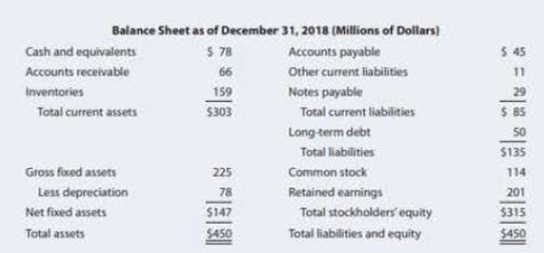Chapter 4, Problem 24PFundamentals of Financial Manageme...

15th Edition
Eugene F. Brigham + 1 other
ISBN: 9781337395250

Solutions

Chapter
SectionFundamentals of Financial Manageme...

15th Edition
Eugene F. Brigham + 1 other
ISBN: 9781337395250
Textbook ProblemIncome Statement for Year Ended December 31, 2018 (Millions of Dollars) Net sales $795.0 Cost of goods sold 660.0 Gross profit$135.0 Selling expenses 73.5 EBITDA $61.5 Depreciation expenses 12.0 Earnings before interest and taxes (EBIT)$ 49.5 Interest expenses 4.5 Earnings before taxes (EBT) $45.0 Taxes (40%) 18.0 Net income$ 27.0 a. Calculate the ratios you think would be useful in this analysis. b. Construct a DuPont equation, and compare the company’s ratios to the industry average ratios. c. Do the balance-sheet accounts or the income statement figures seem to be primarily responsible for the low profits? d. Which specific accounts seem to be most out of line relative to other firms in the industry? e. If the firm had a pronounced seasonal sales pattern or if it grew rapidly during the year, how might that affect the validity of your ratio analysis? How might you correct for such potential problems?

a.

Summary Introduction

To determine: The ratios that would be useful in this analysis.

Current Ratio:

Current ratio is a part of liquidity ratio, which reflects the capability of the company to payback its short-term debts. It is calculated based on the current assets and current liabilities that a company possess in an accounting period.

Ratio Analysis:

Ratio is used to compare two arithmetical figures. In case of the ratio analysis of the company, the financial ratios are calculated. The financial ratios examine the performance of the company and is used in comparing with other same business. It indicates relationship of two or more parts of financial statements.

Explanation

Given information:

Current assets are $303. Current liabilities are$85.

The formula to calculate current ratio is as follows:

Current Ratio=Current AssetsCurrent Liabilities

Substitute $303 for current assets and$85 for current liabilities.

Current Ratio=$303$85=3.56times

Thus, current ratio is 3.56 times.

Fixed Assets Turnover Ratio:

It reflects the efficiency of the company to utilize fixed asset to increase sale.

Given information:

Sales are $795. Net fixed assets are$147.

The formula to calculate fixed assets turnover is as follows:

Fixed Assets Turnover=Total Sales Net Fixed Assets

Substitute $795 for total sales and$`147 for fixed assets in above formula.

Fixed Assets Turnover=$795$147=5.40 times

Thus, fixed assets turnover is 5.40 times.

Debt-to-Capital Ratio:

It is percentage of total capital that is financed by the borrowed fund. Borrowed fund includes short and long-term debts. Operating debt like account payable, accrual are not considered.

Given information:

Total debt is $79 (working note). Equity is$315 (working note).

The formula of Debt-to-capital ratio is as follows:

Debt-to-Capital Ratio=Total DebtDebt+Equity×100

Substitute $79 for total debt and$315 for equity in above formula.

Debt-to-Capital Ratio=$79$79+$315×100=$79$394×100=20.05% Thus, debt-to-capital ratio is 20.05%. Working notes: Compute total debt. Given information: Long-term debt is$50.

Notes payable is $29. Calculation of total debt of the company is as follows: Total Debt=Long-term Debt+Notes Payable=$50+$29=$79 (1)

Thus, total debt is $79. Compute the total value of the common equity is as follows: Given information: Common stock is$114.

Retained earnings are $201. Formula to calculate common equity is as follows: Common Equity=Common Stock+Retained Earnings Substitute$114 for common stock and $201 for retained earnings. Common Equity=$114+$201=$315 (2)

Thus, common equity is $315. Total Assets Turnover Ratio: It indicates how effectively the asset of a company is utilized. Total asset is the sum of current assets and fixed assets. Given, Total sales is$795

Total assets are $450. Formula to calculate total assets turnover is, Total Assets Turnover=Total SalesTotal Assets Substitute$795 for total sales and $450 for total assets. Total Assets Turnover=$795$450=1.76 times Thus, total assets turnover is 1.76 times. Times-Interest Earned Ratio: It is the type of solvency ratio that indicates the capability of business to repay interest and provide debt related services. Given, Earnings before interest and tax (EBIT) are$49.5.

Interest expense is $4.5. Formula to calculate times interest earned is as follows: Times Interest Earned=EBITInterest Expenses Substitute$49.5 for EBIT and $4.5 for interest expense. Times Interest Earned=$49.5$4.5=11% Thus, the times interest earned ratio is 11%. Profit Margin Profit margin is the ratio net income of the company and total sales. It is one of the profitability ratios. Given information: Net income is$27.

Sales are $795. Formula to calculate profit ratio is as follows: Profit Ratio=Net IncomeSales×100 Substitute$27 for net income and \$795 for sales in above formula

b.

Summary Introduction

To determine: DuPont equation and comparison of company’s ratio with industry average ratio.

Du Pont Equation:

Among all ratios, return on equity is very common. It shows the value of the firm. Improvement in the ROE is considered as valued addition to the firm. ROE can be linked with other ratios. Analysis of such ratios will indicate proper reason for change in ROE. The combination is known as Du Pont equation, which is shown below.

ROE =Net IncomeCommon Equity=Net IncomeTotal Assets×Total AssetsCommon Assets=Net IncomeSales×SalesTotal Assets×Total AssetsTotal Common Equity=Profit Margin× Total Assets Turnover Ratio×Equity Multiplier

c.

Summary Introduction

To identify: The balance sheet accounts or income statement figures are primarily responsible for the low profits or not.

d.

Summary Introduction

To identify: The specific accounts seems to be most out of line comparative to other companies in the same industry.

(e)

Summary Introduction

To identify: The validity of the ratio analysis if the firm had marked seasonal sales pattern or if the sales grew rapidly during the year and the way of correcting potential problems.

Still sussing out bartleby?

Check out a sample textbook solution.

See a sample solution

The Solution to Your Study Problems

Bartleby provides explanations to thousands of textbook problems written by our experts, many with advanced degrees!

Get Started

Find more solutions based on key concepts